# Power Measurements

Summary of the Power Measurements components available in Schematic Editor.

This sub-category provides several Power measurement components. Their descriptions and supported features are given in Table 1.

Table 1. Power measurement components in the Signal Processing category of the Schematic Editor core library
Component Description Features Help file
supported input types supported output types vector support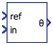Phase Difference

Calculates phase difference between the input signals. real real no Phase Difference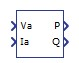Single Phase Power Meter

Computes active and reactive power for single phase systems. Inputs are the instant voltage and current. real real no Single Phase Power Meter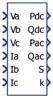Power Meter

Computes active and reactive power (both instantaneous and average), apparent power, and power factor for three phase systems. real real no Power Meter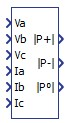Positive, Negative and Zero Power Meter

Calculates the positive, negative, and zero power sequence for input signals.

For successful operation of the component block, 1/(frequency*execution rate) must be an integer greater than 4.

real real no Positive Negative Zero Power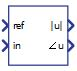Single Phase Phasor

Calculates phasor (magnitude and angle) of the input signal. real real no Single phase phasorThree Phase Phasor

Calculates phasors (magnitude and angle) of the input signals. real real no Three Phase Phasor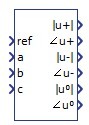Symmetrical Components

Gives phasors of symmetrical components of the input signals.

For successful operation of the component block, 1/(frequency*execution rate) must be an integer greater than 4.

real real no Symmetrical Components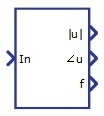Harmonic Analyzer

Calculates amplitude, phase, and frequency of the chosen harmonics. Outputs are scalars if the Hamonic order value is scalar, while if the Hamonic order value is a Python list, outputs will be vectors. The component consists of two parts, with one part working on a faster execution rate than the other. The slower part is used to calculate FFT. real real no Harmonic Analyzer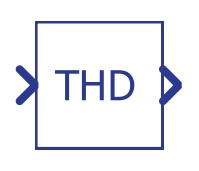THD Measurement

Calculates total harmonic distortion of the observed signal, based on the THD+N approach.

The component consists of two components; the first one is buffer which has to work on a faster execution rate, and the second one has to work on a much slower execution rate. The component with a slower execution rate is used for FFT calculation and other needed arithmetic.

real real no THD (Total Harmonic Distortion) Measurement